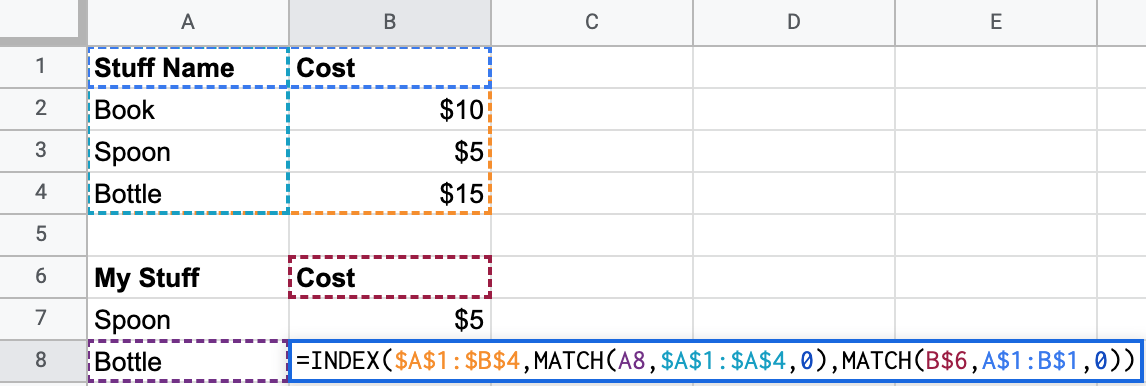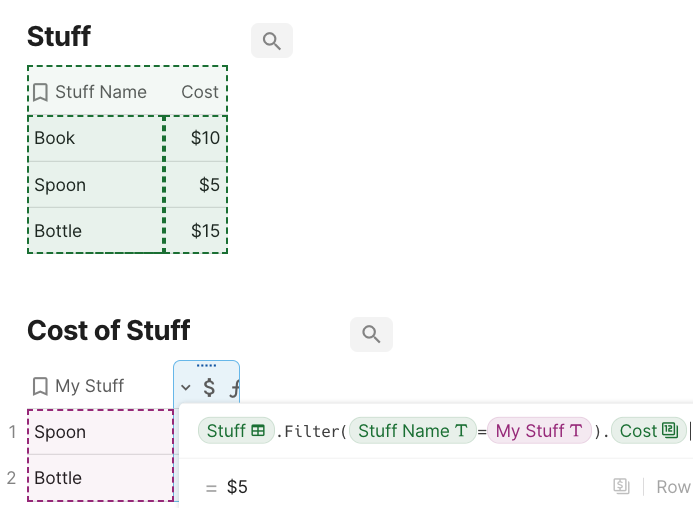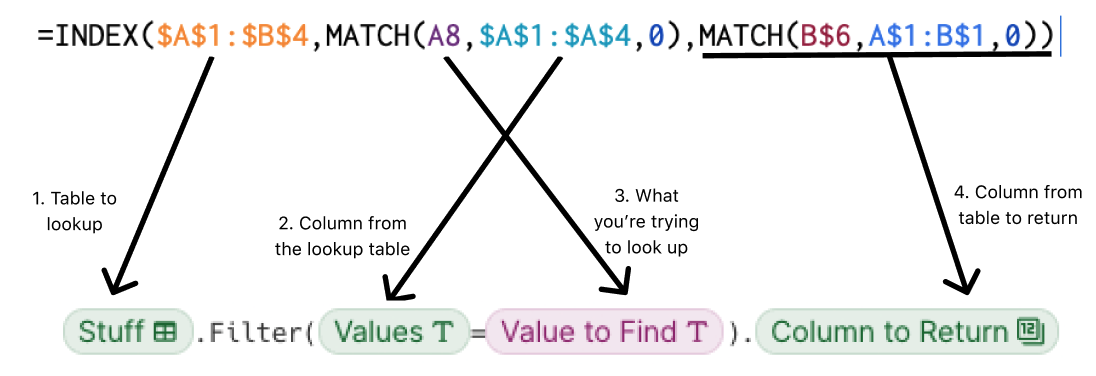Share
Explore

#Index(Match)Index(Match)### How to do the equivalent of a Index(Match) in CodaCoda### Formula side-by-side comparisonIndex(Match)
0
Parameter
Index(Match) in Excel/GSheets
FILTER in Coda
1
1. Table Array to Match
Table array with the relevant data you want to match
Name of the table (e.g. “Stuff”)
2
2. Column from the Index table
Range of data you want to match to get the row Index
Name of the column in the Index table you want to Match stuff (e.g. “Values”)
3
3. What you’re trying to Match
Usually a cell reference or a hard-coded value (e.g. A7 or “Spoon”) That you are trying to match in the given range(in this case you we are trying to match cell A8 within the range (A1:A4))
Name of the column you want Match in the index table (e.g. “Value to Find”)
4
4. Column from the Index table to return
Usually a number indicating how many columns to the right of the first column in the lookup table you want to return
Name of the column you want to return from the Index table (e.g. “Column to Return” column from the “Stuff” table)
There are no rows in this table### High-level differences between Index(Match) and FILTER

Dot Operator Vs. Nested Formula’s: In Excel and Sheets You need to nest the match formula within the index formula to get the desired results. In Coda, you can use dot operators to eliminate the need to nest formula’s.
Readability: Another benefit of Coda formulas is the the readability of the standalone formula. It’s vey hard for an excel user to translate the index(match) formula into a sentence unless they look at the underlying data. The filter formula above translates to, “Filter the “Stuff” table where the “Value to Find” = the “values” Column in the “Stuff” table(you can tell this by the same pill color) and return the value in “Column to Return.”